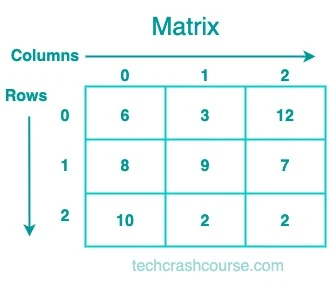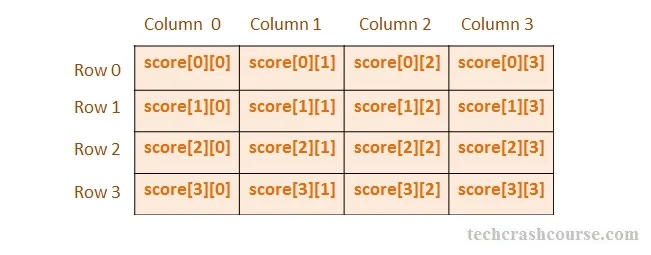# C++ Multidimensional Arrays

C++ programming language supports multi dimensional Arrays. Multidimensional arrays can be described as "arrays of arrays". A multidimensional array in C++ is an array of arrays, extending the concept of a one-dimensional array to handle more complex data structures.

The most common type of multidimensional array is the two-dimensional array, often used to represent matrices. These arrays offer a structured and powerful way to represent complex data structures, such as matrices and grids. For Example, a two dimensional array:

```int matrix;
```

can be imagined as a table of 12 elements arranged in 3 rows and 4 columns. First dimension represents the number of row and second dimension represent number of vertical columns.

• Multi dimensional arrays are array of arrays.
• Multi dimensional arrays have more than one subscript variables.
• Multi dimensional array is also called as matrix.

## Practical uses of Multidimensional Arrays

• Image Processing : Matrices are widely used in image processing, where each pixel's color intensity can be represented by a matrix, and various operations like filtering and transformations are performed.

• Linear Algebra : Matrices play a central role in linear algebra, representing systems of linear equations, transformations, and eigenvalue problems.

• Graph Representations : Adjacency matrices are used to represent graphs, where each element indicates the presence or absence of an edge between two vertices.

• Machine Learning : In machine learning, matrices are extensively used to represent datasets, weights in neural networks, and coefficients in regression models.

## Multidimensional Array Declaration

data_type array_name[size1][size2]...[sizeN];

Above statement will declare an array of N dimensions of name array_name, where each element of array is of type data_type. The maximum number of elements that can be stored in a multi dimensional array array_name is size1 X size2 X size3...sixeN.

For Example :
```Declaration of two dimensional integer array
int maze;
Declaration of three dimensional character array
char rubix;
```

## Representation of a Matrix

A matrix is a two-dimensional array of numbers, symbols, or expressions, organized in rows and columns. The size of a matrix is determined by the number of rows and columns it contains. Commonly denoted as m×n, where m is the number of rows, and n is the number of columns. A matrix can be represented as a two-dimensional array of elements. The number of rows and columns in the matrix is specified when it is created. The elements of the matrix can be of any data type, such as integers, floats, or characters.

Here is an example of a 3x3(3 rows and 3 columns) matrix of integers:Key characteristics of matrices include:

• Row and Column Indices : Elements in a matrix are identified by their row and column indices.

• Order or Size : The order of a matrix is given by the number of rows and columns (e.g., 3×4, for a matrix with 3 rows and 4 columns).

• Equality : Two matrices are equal if they have the same order and corresponding elements are equal.

## Multidimensional Array Initialization

Initialization of Two Dimensional Array

Two dimensional arrays can be initialized by specifying elements for each row inside curly braces. The following declaration initializes a two dimensional matrix of 4 rows and 3 columns.

```int matrix = {
{1, 2, 3},    /* Initialization of first row */
{4, 5, 6},    /* Initialization of second row */
{7, 8, 9},    /* Initialization of third row */
{10, 11, 12}, /* Initialization of fourth row */
};
```

Similarly, we can initialize any multi dimensional array.
A two dimensional matrix can be initialized without using any internal curly braces as follows:

```int matrix = {1, 2, 3, 4, 5, 6, 7, 8, 9, 10, 11, 12};
```

In above declaration, compiler will assign first four values(1, 2, 3 and 4) to first row of matrix and within a row it will populate elements from left to right. Then next four elements to second row and so on.

Initialization of Three Dimensional Array

Similarly, we can initialize any multi dimensional array.
Similar to two dimensional array, we can also initialize 3 dimensional array as follows :

```int rubix = {4, 6, 1, 0, 6, 12, 3, 10, 0, -4, 8, 9};
```

## Two Dimensional Array in C++

Two dimensional arrays are most common type of multi dimensional array in C++. A two dimensional array in C++ language is represented in the form 2D matrix having rows and columns.

• A two dimensional matrix is an array of one dimensional arrays.
• Two dimensional array requires two subscript variables or indexes.
• One subscript represents the row index while other represent column index of an element in matrix.
• Elements of a two dimensional array are stored in contiguous memory location. First all elements of first row are store then all elements of second row and so on.Any element in two dimensional matrix is uniquely identified by array_name[row_Index][column_Index], where row_index and column_index are the subscripts that uniquely specifies the position of an element in a 2D matrix.
An element in second row and third column of an two dimensional matrix whose name is board is accessed by board. Like single dimensional array, indexing starts from 0 instead of 1.
We can declare a two dimensional matrix of R rows and C columns as follows:

```data_type array_name[R][C];
```
For Example :

A two dimensional integer matrix of 3 rows and 3 columns can be declared as

```int score;
```

## C++ Two Dimensional Array Example program

```#include <iostream>
using namespace std;

int main(){
// Two dimentional array declaration
int matrix;
int rowCounter, colCounter, rows, cols, sum=0;

cout << "Enter size of a matrix\n";
cin >> rows >> cols;
// Populating elements inside matrix
cout << "Enter elements of a matrix\n";
for(rowCounter = 0; rowCounter < rows; rowCounter++){
for(colCounter = 0; colCounter < cols; colCounter++){
cin >> matrix[rowCounter][colCounter];
}
}
// Accessing all elements of matrix to calculate their sum
for(rowCounter = 0; rowCounter < rows; rowCounter++){
for(colCounter = 0; colCounter < cols; colCounter++){
sum += matrix[rowCounter][colCounter];
}
}

cout << "Sum of all elements = " << sum;

return 0;
}
```

Output
```Enter size of a matrix
3 3
Enter elements of a matrix
1 2 3
3 4 5
6 7 8
Sum of all elements = 39
```
In above program, we first take number of rows and columns as input from user. Then using two for loops we take matrix elements input from user and store it in 2D array named matrix. To find the sum of each element of matrix we traverse the matrix row wise using two for loop.

## Working with Three-Dimensional Arrays in C++

While two-dimensional arrays are common, C++ supports arrays with more than two dimensions. A three-dimensional array is an array of arrays of arrays.
• Syntax of 3D Array Declaration
```// Syntax of 3D array declaration
dataType arrayName[xSize][ySize][zSize];
```
Here, xSize, ySize, and zSize represent the sizes along the three dimensions.
```int cube;
```
This declares a three-dimensional array named cube with sizes 2x3x4.

• Accessing Elements in a 3D Array : Accessing elements in a three-dimensional array requires specifying indices for each dimension.
```int cube = {
{
{1, 2, 3, 4},
{5, 6, 7, 8},
{9, 10, 11, 12}
},
{
{13, 14, 15, 16},
{17, 18, 19, 20},
{21, 22, 23, 24}
}
};

// Accessing elements in a 3D array
int element = cube;
// Retrieves the element at x=1, y=2, z=3 (value: 24)
```
Here, cube retrieves the value at the specified coordinates.

## Dynamic Memory Allocation for Multidimensional Arrays

For dynamic sizing, multidimensional arrays can be allocated using pointers and dynamic memory allocation.
• Dynamic Allocation of a 2D Array :
```// Dynamic allocation of a 2D array
int** dynamicMatrix = new int*;
for (int i = 0; i < 3; ++i) {
dynamicMatrix[i] = new int;
}
```
Here, dynamicMatrix is a dynamically allocated 3x3 matrix.

• Dynamic Allocation of a 3D Array :
```// Dynamic allocation of a 3D array
int*** dynamicCube = new int**;
for (int i = 0; i < 2; ++i) {
dynamicCube[i] = new int*;
for (int j = 0; j < 3; ++j) {
dynamicCube[i][j] = new int;
}
}
```
This example demonstrates dynamic allocation for a 2x3x4 cube.

### Conclusion

Multidimensional arrays in C++ open up a vast array of possibilities for representing complex data structures and solving diverse computational problems. By mastering the syntax, operations, and best practices associated with multidimensional arrays, you empower yourself to tackle a wide range of programming challenges.